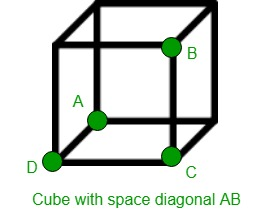# Volume of cube using its space diagonal

Given the length of space diagonal of a cube as d. The task is to calculate the volume occupied by the cube with the given length of space diagonal. Space diagonal is a line connecting two vertices that are not on the same face.Examples:

Input: d = 5
Output: Volume of Cube: 24.0563

Input: d = 10
Output: Volume of Cube: 192.45


## Recommended: Please try your approach on {IDE} first, before moving on to the solution.

Volume of cube whose space diagonal is given:Proof:

Let d = the length of diagonal |AB| and
let a = the length of each side of the cube.
Pythagorus #1 in triangle ACD:Pythagorus #2 in triangle ABC:Now we can solve for a in terms of d:This means that the volume V is:Below is the required implementation:

## C++

 // C++ program to find the volume occupied  // by Cube with given space diagonal  #include  using namespace std;     // Function to calculate Volume  float CubeVolume(float d)  {      float Volume;         // Formula to find Volume      Volume = (sqrt(3) * pow(d, 3)) / 9;         return Volume;  }     // Drivers code  int main()  {         // space diagonal of Cube      float d = 5;         cout << "Volume of Cube: "          << CubeVolume(d);         return 0;  }

## Java

 // Java program to find the volume occupied   // by Cube with given space diagonal      public class GFG{             // Function to calculate Volume       static float CubeVolume(float d)       {           float Volume;                  // Formula to find Volume           Volume = (float) (Math.sqrt(3) * Math.pow(d, 3)) / 9;                  return Volume;       }              // Drivers code       public static void main(String []args)      {                  // space diagonal of Cube           float d = 5;                  System.out.println("Volume of Cube: " + CubeVolume(d));              }          // This code is contributed by Ryuga      }

## Python3

 # Python 3 program to find the volume occupied  # by Cube with given space diagonal  from math import sqrt, pow    # Function to calculate Volume  def CubeVolume(d):         # Formula to find Volume      Volume = (sqrt(3) * pow(d, 3)) / 9        return Volume     # Drivers code  if __name__ == '__main__':         # space diagonal of Cube      d = 5        print("Volume of Cube:",'{0:.6}' .                   format(CubeVolume(d)))     # This code is contributed  # by SURENDRA_GANGWAR

## C#

 // C# program to find the volume occupied   // by Cube with given space diagonal   using System;     public class GFG{             // Function to calculate Volume       static float CubeVolume(float d)       {           float Volume;                  // Formula to find Volume           Volume = (float) (Math.Sqrt(3) * Math.Pow(d, 3)) / 9;                  return Volume;       }              // Drivers code       public static void Main()      {                  // space diagonal of Cube           float d = 5;                  Console.WriteLine("Volume of Cube: {0:F4}" , CubeVolume(d));              }          // This code is contributed by mits      }

## PHP

 

Output:

Volume of Cube: 24.0563


Time Complexity: O(1)

Attention reader! Don’t stop learning now. Get hold of all the important DSA concepts with the DSA Self Paced Course at a student-friendly price and become industry ready.

My Personal Notes arrow_drop_upCheck out this Author's contributed articles.

If you like GeeksforGeeks and would like to contribute, you can also write an article using contribute.geeksforgeeks.org or mail your article to contribute@geeksforgeeks.org. See your article appearing on the GeeksforGeeks main page and help other Geeks.

Please Improve this article if you find anything incorrect by clicking on the "Improve Article" button below.

Article Tags :
Practice Tags :

Be the First to upvote.

Please write to us at contribute@geeksforgeeks.org to report any issue with the above content.